# PyImageSearch University: You can master Computer Vision, Deep Learning, and OpenCV

I strongly believe that if you had the right teacher you could master computer vision and deep learning.

Do you think learning computer vision and deep learning has to be time-consuming, overwhelming, and complicated? Or has to involve complex mathematics and equations? Or requires a degree in computer science?

That’s not the case.

All you need to master computer vision and deep learning is for someone to explain things to you in simple, intuitive terms. And that’s exactly what I do. My mission is to change education and how complex Artificial Intelligence topics are taught.

Welcome to PyImageSearch University, the most comprehensive computer vision, deep learning, and OpenCV course online today. Here you’ll learn how to successfully and confidently apply computer vision to your work, research, and projects. Join me in computer vision mastery.

Created by: Adrian Rosebrock, PhD •Last updated: 9/2021 •Languages: English

4.84 (128 Ratings) • 10,597 Students Enrolled
##### What you'll be able to do...
• Successfully complete your computer vision and deep learning projects
• Land a job in the Artificial Intelligence field
• Apply computer vision and deep learning to your job and workplace
• Finish your MSc or PhD thesis
• Perform novel research and publish paper in a reputable AI journal
• Learn computer vision and deep learning, and then teach your high school or college students
• Understand computer vision and deep learning, and launch a business in the AI space
• Finish that AI project you are hacking on over nights and weekends
##### Requirements

In order to be successful in PyImageSearch University, you need the following:

• Understanding of Python basics
• Internet connection
• Windows, macOS, Linux, or Raspbian (all major operating systems supported)
• Free Gmail/Google account to run pre-configured Jupyter Notebooks in Colab (optional)
• A desire to learn
##### Course description

PyImageSearch University is a comprehensive set of self-paced courses for developers, students, and researchers who are ready to master computer vision, deep learning, and OpenCV. Inside this course you’ll learn how to successfully and confidently apply computer vision to your work, research, and projects.

Unlike other online courses, which are created once and never updated, leaving you with stale, out-of-date information, I keep PyImageSearch University up-to-date by releasing a brand new class every month!

Releasing a new class every month ensures you can keep up with the state-of-the-art in computer vision and deep learning, learn new algorithms and techniques, and:

• Successfully complete your projects at work
• Perform novel research (and publish papers)
• Launch your next company in the Artificial Intelligence space

• Detailed video tutorials for every lesson
• High-quality, well documented source code with line-by-line explanations (ensuring you know exactly what the code is doing)
• Jupyter Notebooks that are pre-configured to run in Google Colab with a single click
• Support for all major operating systems (Windows, macOS, Linux, and Raspbian)

PyImageSearch University is without a doubt the most complete, comprehensive computer vision education online inside. I’ll see you inside.

CEO, PyImageSearch.com

##### Trusted by members of top artificial intelligence companies, schools, and organizations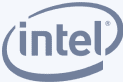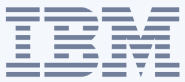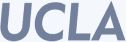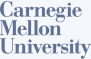##### Who this course is for:

If any of these descriptions fit you, rest assured, PyImageSearch University is designed for you.

• You are a computer vision practitioner that utilizes deep learning and OpenCV at your day job, and you’re eager to level-up your skills.
• You’re a developer who wants to learn computer vision/deep learning, complete your challenging project at work, and stand out from your coworkers (and land that big promotion).
• You are a college student who needs help with your homework, completing your final graduation project, or you simply want more than what your university offers.
• You are a researcher or scientist looking to apply computer vision and deep learning techniques to your research (and publish a paper).
• You have experience with machine learning and want to learn more about deep learning and neural networks.
• You are an entrepreneur studying computer vision/deep learning so you can launch your next business in the Artificial Intelligence space.
• You are a “computer vision hobbyist” who wants to successfully complete that project you are hacking on over nights and weekends.
• You’re a PyImageSearch reader that wants access to centralized repos containing high-quality, well documented source code, pre-trained models, image datasets, etc. for all 400+ tutorials on PyImageSearch.com.
• You prefer running code examples with Jupyter Notebooks in Google Colab — my notebooks are pre-configured and ready to run in Google Colab with only a single click.
• You learn best through video tutorials — PyImageSearch University includes video guides for every single lesson.
##### 25 Certificates of Completion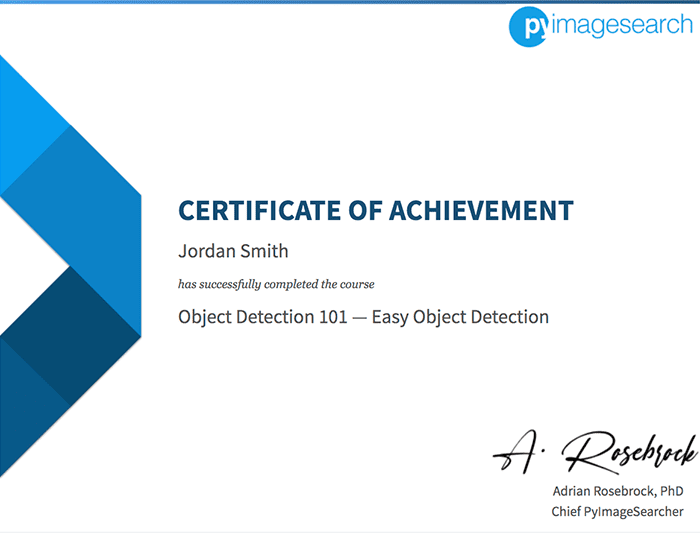We don’t offer just one Certificate of Completion like most online courses. Instead, we offer a certificate for each of the 25 courses inside PyImageSearch University.

And since a brand new course is released every month, that means each month you receive…

• A brand new course
• A new set of lessons
• A new set of quizzes
• A new final exam
• And another opportunity to demonstrate your computer vision and deep learning knowledge to the world

PyImageSearch graduates have gone on to:

PyImageSearch University is your chance to join them in computer vision and deep learning mastery.

##### PyImageSearch University syllabus

25 Courses •128 Classes •37h 19m 02s Lectures

• Foundations of Machine Learning
ML with Python path

4h 12m 19s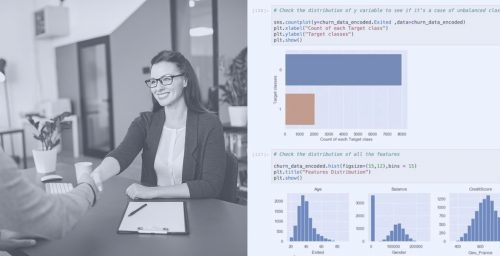Broad Types of ML Problems Part-1 (02:23)

Broad Types of ML Problems Part-2 (04:12)

Broad Types of ML Problems Part-3 (04:13)

Industrial Applications of ML Part-2 (08:43)

What ML Can and Cannot Do (08:23)

Data Science vs ML vs AI vs Deep Learning vs Statistical Modeling (08:28)

Introduction to ML Project Workflow (06:10)

Discover (05:19)

Design (04:16)

Develop (02:37)

Testing (04:15)

Deploy (04:16)

Interpreting ML Models (03:45)

Interpreting ML Models Part-1 (06:02)

Interpreting ML Models Part-2 (03:56)

How to Validate ML Models (06:16)

Need for Validation Sample (04:19)

ML Terminology You Need to Know - Part 1 - Supervised vs Unsupervised Learning (06:34)

ML Terminology You Need to Know - Part 2 - Independent vs Dependent Variables (04:49)

ML Terminology You Need to Know - Part 3 - More Terms (04:27)

What is Ensemble Learning (05:12)

Reinforcement Learning Intuition (05:15)

Basic Statistical Concepts Part-1 (07:33)

Basic Statistical Concepts Part-2 (04:47)

Role of Significance Tests (04:17)

Summary

Assignments/challenges

Certificate of Completion

• Numpy for Data Science
ML with Python path

3h 07m 38s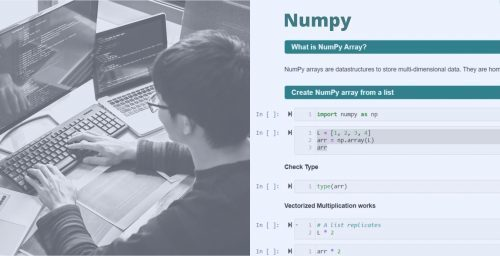Python Setup Local Installation (10:37)

Inspecting Arrays (05:24)

Why DataTypes Matter (08:43)

Import and Export Data (07:33)

Missing Data (03:47)

Extracting Specific Items (12:50)

Random Numbers (11:22)

Set Operations (06:17)

Statistical Summaries (06:28)

Reshaping and New Axis (08:15)

Sequences and Repetitions (5:30)

Mesh Grid (04:06)

Concatenate and Split (06:11)

At and iat (04:12)

Mini Challenge (01:32)

Filtering Data Sorting Methods (08:30)

Searchsorted and Bisect (06:21)

Handling Dates (07:04)

Sorting (02:42)

Vectorization (05:24)

Apply Along (06:13)

Universal Functions (UFuncs) (03:37)

Interpolation (06:48)

Fitting Polynomials (09:24)

Matrix Operations(10:43)

Solving Linear Equations (02:33)

Closing Remarks (01:47)

Summary (02:04)

Assignment/ Challenges

Certificate of completion

• Pandas for Data Science
ML with Python path

6h 34m 17s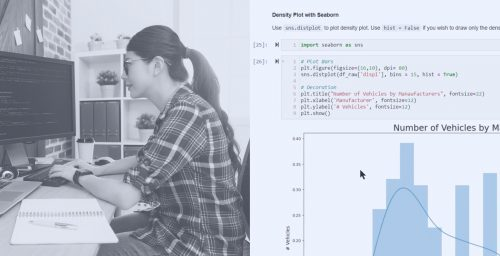Creating Dataframe (08:37)

Mini Challenge (00:48)

Series (07:24)

Mini Challenge (02:43)

Python Setup Local Installation (09:47)

Inspecting Dataframes (04:50)

Renaming Columns (04:22)

Pandas Summary (03:17)

Essential Operations (04:28)

Display Options (02:15)

Extracting Specific Part of Data - Part 1 (5:30)

Extracting Specific Part of Data - Part 2 (02:06)

Mini Challenge (01:11)

At and iat (04:12)

Mini Challenge (01:32)

Filtering Data That Satisfy Conditions (05:30)

Membership Filtering (04:21)

Query and Eval (04:04)

Sorting (02:42)

Map and applymap (07:24)

Apply a function rowwise or columnwise (7:02)

Scaling and Standardization (05:05)

Make Index as a Dataframe Column (02:09)

Discretization and Binning (05:26)

Random Sampling (05:13)

Dummy Variables (02:03)

Categorical Data Part-1 (3:32)

Categorical Data Part-2 (03:07)

Efficiently Read Data From Multiple Files (05:30)

Group by Mechanism (3:10)

Mini Challenge (02:04)

Iterating Between groups (3:02)

Transform (04:05)

Reshaping and Pivoting Data (02:09)

Cross Tabulation (02:26)

Pivoting (04:13)

Wide to Long and Back (07:03)

Joining Dataframes (04:32)

Types of Joins (06:07)

Concatenating Dataframes (2:08)

Representing Missing Values (05:07)

Threshold Based Dropping (02:30)

Approaches To Filling Missing Data (04:18)

Interpolation (06:18)

Compressed File Formats (4:02)

Sparse Datatype (03:05)

Combining Categories (03:09)

Split Contents of a Column (01:26)

Insert Column at Specific Location (02:13)

Select using both Position and Lab (04:03)

Styling Dataframes (02:32)

Comprehensive Profile Report (04:07)

Interactive Plots (2:08)

Third Party Data (04:07)

Interactive Data Analysis (05:30)

Optimizing Dataframes (05:18)

Efficient File Formats (2:02)

HDF5 (06:05)

Chunking (04:09)

Faster Pandas (01:13)

Numba (07:05)

Modin (06:13)

Swifter (05:03)

Vaex (13:32)

Cython (07:07)

Cythonize Pandas Code (6:08)

Cythonize Apply(06:07)

Matplotlib Part 1 - Getting Started (07:30)

Matplotlib Part 2 - Plot Components (07:18)

Matplotlib Part 3 - Subplots (07:18)

Matplotlib Part 4 - Annotations (05:02)

Dual Axis Line Plots (07:05)

Bar Charts (06:09)

Histogram and Density Plots (02:26)

Regression Plots (06:13)

Pair Plots (07:13)

Summary (02:45)

Assignment/ Challenges

Certificate of completion

• Restaurant Visitor Forecasting Project
ML with Python path

4h 58m 07s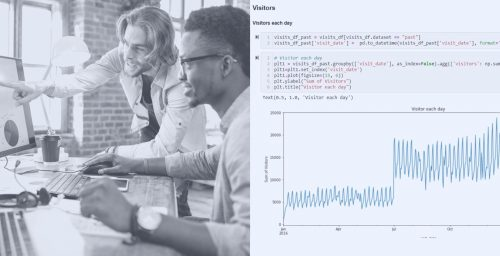Data Overview (04:46)

Basic Data Stats (08:33)

Feature Engineering - Domain Specific (04:43)

Feature Engineering - Interaction Features (03:54)

Exploratory Data Analysis Part 2 (04:34)

ANOVA Concept - Intuition (10:07)

ANOVA Concept - Maths (13:22)

Significance Tests (08:23)

Label Encoding (02:04)

Data Preprocessing for Model Building (04:08)

Feature Encoding Approaches (08:43)

Evaluation Metrics (05:43)

Introduction to Time Series Modeling (01:09)

Time Series and Stationarity Concepts (11:09)

ACF and PACF (08:02)

Introduction to ARIMA Modeling (05:26)

AR and MA Models - Part 1 (06:55)

AR and MA Models - Part 2 (06:23)

Auto Arima Concept (03:45)

Strategy 1 - ARIMA Forecasting Demo (07:34))

Strategy 1 - ARIMA Forecasting Demo - Part 2 (08:55)

Durbin Watson Statistic (03:08)

Strategy 2 - Auto ARIMA (08:12)

Strategy 3 - AutoARIMA for Genre (09:34)

SARIMA and SARIMAX (06:33)

Strategy 4 SARIMA Demo (06:34)

Strategy 5 SARIMAX Demo (10:18)

Exogenous Variables Deepdive Part 1 - Time Based and Demographics (05:03)

Exogenous Variables Deepdive Part 2 - Qualitative and Promotions (05:04)

Exogenous Variables Deepdive Part 3 - Series decomposition and LifeCycle (12:22)

Exogenous Variables Deepdive Part 4 - Macro Economic Features (03:34)

Forecast for Unknown Future (02:43)

Prophet Forecasting Demo (08:49)

New Plan of Attack (03:56)

XGBoost Demo (08:34)

CatBoost (05:34)

CatBoost Demo (07:23)

Hyper Parameters and Tuning (07:12)

Cohorted Ensembles (07:45)

Cohorted Ensembles Demo (04:34)

Final Words (2:45)

Assignment/ Challenges

Certificate of completion

• Microsoft Malware Detection Project
ML with Python path

4h 52m 21s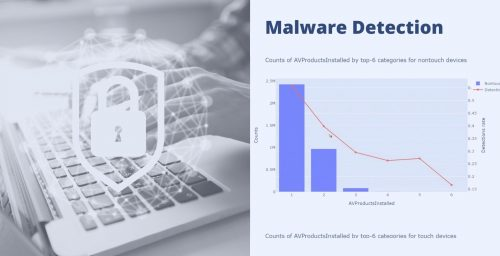Problem Description (5:00)

Complete Industry Dataset

Python Setup - Google Colab (05:06)

Python Setup - Local Installation (10:02)

Optimize Memory Usage (11:19)

Understand The Data (8:00)

Data Preprocessing for EDA (7:00)

Need for EDA (5:39)

Exploratory Data Analysis Part 1 (14:00)

Exploratory Data Analysis Part 2 (13:23)

Chi Squared Test Theory and Maths (18:45)

Chi Square Test and Odds Ratio Demo (09:34)

ANOVA Concept - Intuition (10:23)

ANOVA maths (06:05)

ANOVA Demo (08:00)

Feature Engineering - Domain Specific (08:00)

Feature Encoding Approaches (08:00)

Feature Encoding Demo (08:34)

Data Processing for Model Building (05:23)

Confusion Matrix and Evaluation Metrics (10:19)

Concordance and Discordance (09:34)

Precision Recall Curve (03:34)

Evaluation Metrics Demo (05:09)

Decision Trees and Improvements (17:09)

Random Forests (09:00)

XGBoost (07:09)

LightGBM (08:55)

Tuning Hyperparameters (07:06)

Feature Importance (11:34)

Feature Importance Demo (02:09)

Final Words (01:08)

Assignment/ Challenges

Certificate of completion

• Credit Card Fraud Detection Project
ML with Python path

5h 11m 17s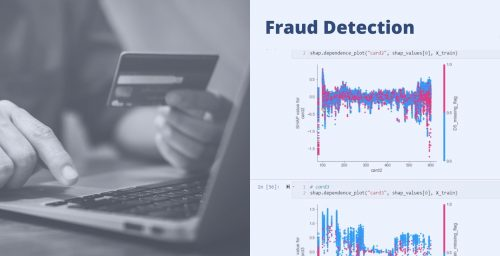Solved notebooks with step-wise explanations

Optimize Memory Usage (05:23)

Data Preprocessing for EDA (04:19)

Exploratory Data Analysis (12:00)

Chi Squared Test Theory and Maths (18:09)

Chi Square Test and Odds Ratio Demo (09:00)

ANOVA Concept - Intuition (10:04)

ANOVA Cancept - Maths (13:03)

ANOVA Demo (07:04)

Feature Engineering (05:09)

Principal Components Analysis (08:02)

Feature Encoding Approaches (8:05)

Feature Encoding Demo (04:09)

Data Preprocessing for Model Building (03:34)

Why XGBoost (04:03)

XGBoost Demo (02:00)

Confusion Matrix and Evaluation Metrics (09:04)

Concordance and Discordance (09:56)

ROC Curve (09:18)

Precision Recall Curve (03:56)

Evaluation Metrics Demo (03:19)

Capture Rates and Calibration Curve (11:45)

Light GBM (08:45)

Light GBM Demo (04:45)

Random Forest Introduction (01:02)

Decision Trees Algorithm (13:49)

Random Forest Classifier Algorithm (06:00)

Extra Trees Algorithm (06:03)

Random Forests Demo (09:09)

Random Oversampler (10:03)

Cost Sensitive Learning with Class Weights (07:34)

Probability Calibration (08:55)

Model Calibration Demo (02:34)

Model Tuning (07:37)

Feature Engineering (05:30)

Feature Importance (11:20)

Feature Importance Demo (03:10)

Partial Dependence and ICE Plots (06:40)

SHAP Interpretations (07:40)

SHAP Demo (05:00)

XGBoost Demo (02:00)

Final Words (02:45)

Assignment/ Challenges

Certificate of completion

• Base-R Programming
ML with R path

6h 48m 18s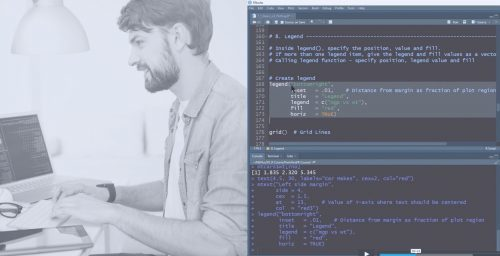Installing R Studio (6:30)

R Studio walkthrough (11:10)

Vectorization (3:47)

Create vector with a single element (10:56)

Create group of elements in a vector (12:10)

Use repetitions and sequence to create a vector fast (6:16)

Random numbers, rounding and sampling (9:00)

Formatting numbers (3:36)

Create subsets (8:04)

Handling Missing Values (10:00)

Binning (9:09)

Operations within a vector (9:12)

Operations between same size vectors (3:44)

Operations between different sized vectors (4:20)

Module Overview (1:30)

Set Operations (5:19)

Making assignments within ifelse (2:48)

Checking existence (1:16)

Nested if-else (3:00)

For loops (4:00)

Writing smarter For loops (2:00)

Break while repeat (5:08)

Memory pre-allocation tactics (5:05)

Why Dates cant just be strings (5:09)

Date operations (1:00

Working with lubridate and anytime (5:00)

Introduction to lists (6:03)

Named list, unlist and more (7:08)

Introduction to Dataframe (3:48)

Creating Dataframe (3:34)

Visual editing (2:25)

Various dataframe operations (11:06)

Inspecting and Rownames (10:23)

Select, delete, subset (7:09)

Saving dataframe to disk (7 :20)

Native RDS files (2:34)

Handling CSV files (2:09)

Xlsx files (2:37)

SAS and Stata files (4 :07)

R datasets, packages and public datasets (11:04)

Useful data summarization function (8:17)

Conditional filtering and missing values (10:45)

Matrix vs dataframe (6:39)

Joining operations for dataframes (9:28)

Pivot and frequency table (11:09)

Grouping and case problem solution (9:29)

Module Overview (2:35)

Base Graphics basics (2 :24)

Scatterplot (1:34)

Legend (1:34)

Saving plot components and challenge (3:34)

Line plot with secondary Y axis (7:39)

Change Par settings (4:24)

Histogram and bar charts (6:34)

Box plot (3:20)

Dot plot and density plot (5:34)

Multiple plots and custom layouts (5:00)

Intro to stringr (5:25))

Sentences, punctuations, strings manipulations (6:45)

Writing effective functions (7:20)

Local and global namespace (12:45)

Debugging R code (9:56)

Error handling (7:40)

Apply Function (3:00)

Apply, sapply, vapply (4:10)

Mapply (3:20)

Summary (2:20)

Assignment/ Challenges

Certificate of completion

• Dplyr for Data Wrangling
ML with R path

36m 47s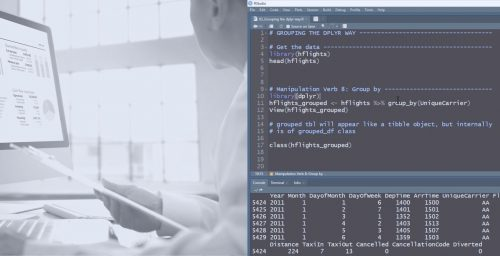T-pipe (01:20)

Compound assignment and exposition pipe (02:10)

Tibble (04:30)

Types of joins in dplyr (03:10)

Joins demo (04:40)

Joins challenges (03:30)

Assignment/ Challenges

• Wrangling Data with Data.Table
ML with R path

50m 41s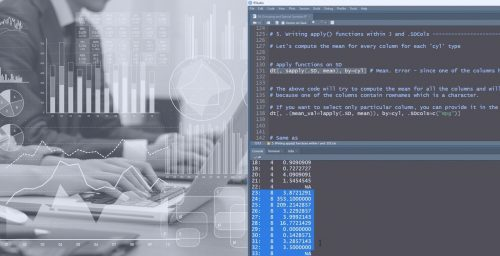Introduction to datatable (03:04)

Creating datatable and importing (07:03)

Datatable syntax (01:04)

Filtering and subsetting (04:05)

Creating new columns (07:06)

Running multiple statements in one (02:07)

Groupby operations (04:40)

Special symbols (04:30)

Applying functions (03:40)

Go faster with keys (10:20)

Fast loops with set function (07:30)

Assignment/ Challenges

Certificate of completion

• GGPlot2 Visualization for Data Analysis
ML with R path

1h 15m 13s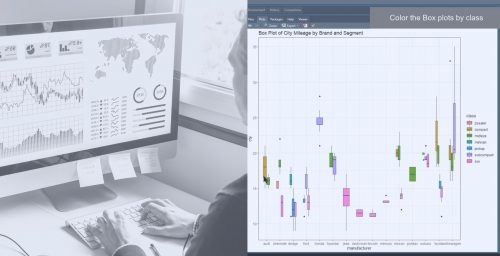Introduction to GGPlot2 (05:04)

Line of Best Fit and Smoothing Lines (01:09)

Adjust X and Y Limits (02:35)

Changing Color of Points (03:45)

Changing Size (01:56)

Removing Legend (01:45)

Color Palette (01:45)

Customize Axis Text (02:45)

Look and Feel (04:10)

Labels and Text (05:56)

Custom Annotations (02:30)

Legend (05:30)

Better Represent Overlapping Points with Jitter and Counts Plot (03:40)

Multiplot with Facets (06:40)

Custom Layout (01:30)

Bar Charts (02:40)

Box Plot and Violin Plots (04:33)

Time Series Plots (06:45)

Multiple Time Series in Same Plot (03:00)

Assignment/ Challenges

Certificate of completion

• Statistical Foundations for ML in R
ML with R path

3h 20m 27s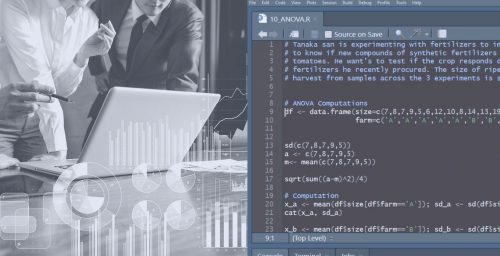Course Overview (01:00)

Statistical Testing (2:09)

Panel Data and Types (3 :34)

Cross Sectional and Pooled Cross Sectional (1:05)

Types of Variables (2:45)

Measures of Central Tendency and Dispersion (11:35)

Demo - Measures of Central Tendency Dispersion (02:46)

Law of Large Numbers (02:33)

Gamblers Fallacy (03 :24)

Normal Distribution (04:45)

Standard Normal Distribution (04:40)

Central Limit Theorem (04:34)

Demo - Central Limit Theorem (04:12)

Standard Error (06:34)

Confidence Intervals Formula (02:34)

Confidence Intervals with Bootstrapping (06:45)

Correlation (13:00)

Demo - Correlation Test (08:45)

Introduction to T-Tests (08:34)

One Sample T-Test (08:44)

One Sample T-Test - R Demo (06:33)

One Sample T-Test - Hand Computation (08:23)

What is Two Sample Paired T-Test (02:44)

Two Sample Dependent T test - Examples of when to use (05:45)

Two Sample Dependent T test - Hand Computation (10:45)

Two Sample Dependent T test - R Demo (08:34)

What is Independent Two Sample T-test (02:55)

Independent Two Sample T test - Examples of when to use (02:23)

Independent Two Sample T test - Hand Computation (08:34)

Independent Two Sample T test - R Demo (03:23)

What is Chi-Squared Test (03:09)

Chi-Squared Test - Examples of When to Use (02:04)

Chi Square Test - Hand Computation (10:22)

Chi-Squared Test - R Demo (03 :44)

What is ANOVA (04:34)

When to Use ANOVA (04:56)

ANOVA Terminologies (04:37)

ANOVA - Hand Computation (05:45)

ANOVA - R Demo (03:27)

Assignment/ Challenges

Certificate of completion

• Regression Modeling in R
ML with R path

5h 07m 13s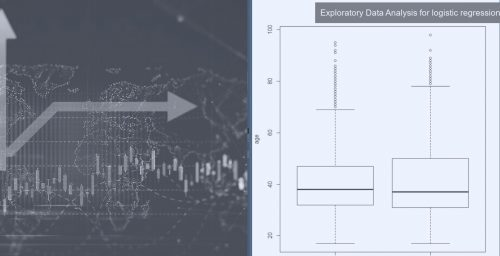Understanding with Practical Examples (02:45)

Examples of Industrial Applications (02:33)

Statistical Modeling vs Machine Learning (02:23)

Graphical Understanding (03:34)

Formulate Line of Best Fit (04:34)

Linear Regression from Scratch using Formula (07:24)

Pre-Model Build Analysis (05:45)

Building and Interpreting Linear Regression Models (05:45)

Problem with R-Squared (02:34)

F-Statistic, AIC, BIC (03:34)

R-Demo (04:34)

Assumptions (18:34)

Problem Statement (03:20)

Handling Missing Values (09:20)

Outlier Analysis (04:27)

Graphical and Statistical Analysis (10:20)

Building Linear Regression (07:00)

Good Model (03:05)

Evaluation Measures (05:00)

Need for Cross Validation (12:03)

Cross Validation Approaches (05:30)

Variable Transformations and Interactions (05:20)

Variance Inflation Factor (04:30)

Cooks Distance for Influential Points (06:10)

Cooks Distance Demo (05:04)

BoxCox and YeoJohnson Transformations (06:20)

Residual Analysis (06:20)

Overcoming Heteroscedasticity (08:20)

Stepwise Regression for Model Search (05:05)

Best Subsets Model Search (06:05)

What Exactly is Gradient Descent (08:30)

Comparing Types of Gradient Descent (03:10)

Stopping Criteria and Scaling (02:20)

Introduction to Logistic Regression (03:40)

One vs Rest Strategy (02:40)

Use Case Examples (04:20)

Math behind Logistic Regression Part 1 (04:20)

Math behind Logistic Regression Part 2 (04:40)

Why Negative Logloss (03:20)

Problem Statement Marketing (01:20)

Demo - EDA for Logit (09:11)

Building Logistic Regression Model (05:33)

Confusion Matrix and Evaluation Metrics (09:30)

Precision Recall Curve (03:20)

Optimal Cutoff Score (05 :10)

The ROC Curve (02:03)

KS Statistic and Gain Curve (11:10)

Concordant and Discordant pairs (06:20)

Approaches to Handle Class Imbalance (06:33)

Cost Sensitive Learning (06:28)

Oversampling (03:17)

Hybrid Sampling (05:40)

Assignment/ Challenges

Certificate of completion

• Caret Package for ML in R
ML with R path

2h 01m 05s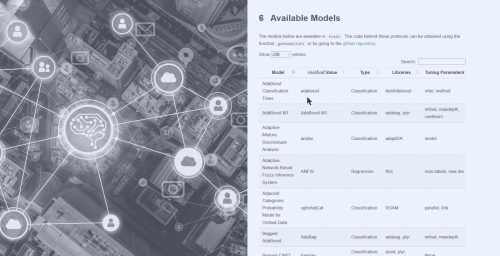Problem Statement (03:08)

Sampling Approaches (08:04)

Missing Value Treatment (07:05)

One Hot Encoding (04:05)

Box Cox Transformation Concept (02:05)

Applying Box Cox and PCA (06:06)

Feature Plots (05:06)

Statistical Analysis (05:06)

Recursive Feature Elimination (RFE) Algorithm (03:05)

Recursive Feature Elimination (RFE Demo) (03:22)

Genetic Algorithms (03:43)

Genetic Algorithms part 2 - Feature Selection (09:33)

Genetic Algorithms Demo (06:34)

Simulated Annealing Concept (06:45)

Simulated Annealing Demo (05:25)

Exploring the ML Models (BIG LIST) (02:14)

Building ML with train function (08:23)

Automatic Hyperparameter Tuning (04:34)

Customize Hyperparameter Search (02:24)

Comparing Adaboost xgboost random forest and svm (02:34)

Tuning Non Default Parameters with Caret (03:45)

Caret Ensembles (04:00)

Assignment/ Challenges

Certificate of completion

• Spacy for NLP
Specialized Courses

4h 27m 25s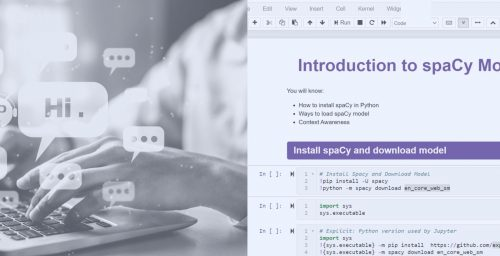Introduction to Spacy Models (08:11)

What Happens Inside Spacy Models (03:02)

Install Spacy and Models (04:05)

Data Structures in Spacy (04:15)

Data structures in Spacy - Make a doc from scratch (03:05)

Data structures in Spacy - nlp.make.doc() (03:09)

String Store (05:06)

How Tokenization Works (04:18)

Create Custom Tokenization Rules (06:19)

Build Custom Tokenizer (04:09)

Named Entity Recognition (07:06)

POS Tagging (05:18)

Dependency Parsing (01:05)

Need for Dependency Parsing (02:13)

Navigating the Parse Tree (02:20)

Noun Chunks (04:13)

Contextual Token Matching (08:15)

Phrase Matching (07:10)

Handling Large Number of Patterns (04:11)

Course Review (01:01)

Understanding Spacy Pipelines (04:00)

How to Apply Particular Component (04:03)

Manipulating the Pipeline (04:14)

Entity Ruler (02:12)

How to Create Custom Pipeline Components (03:12)

Custom Sentence Segmenter (06:07)

Efficient Text Processing Approaches (07:16)

User Defined Attributes (04:07)

Property Extensions (02:21)

Method Extensions (-3:08)

Need for Word Vectors (11:03)

How Word Vectors are Computed (09:08)

Understanding Cosine Similarity (09:22)

Course Review (01:00)

The Training Loop (04:11)

Train a New NER Model from Scratch (07:10)

Training Custom NER Models (05:09)

Training Custom NER Models - Part 2 (03:04)

Training Custom NER Models - Part 3 (03:00)

Training Custom NER Models - Part 4 (3:10)

Course Review (1:00)

Text Classification with TextCat - Part 1 (4:08)

Text Classification with TextCat - Part 2 (2:02)

Text Classification with TextCat - Part 3 (3:10)

Text Classification with TextCat - Part 4 (2:00)

Text Classification with TextCat - Part 5 (3:05)

Text Classification with TextCat - Part 6 (5:15)

Training Customer POS Tagger Model - Part 1 (4:09)

Training Customer POS Tagger Model - Part 2 (5:07)

Course Review (1:00)

Training Custom Intent Parser - Part 1 (5:06)

Training Custom Intent Parser - Part 2 (3:07)

Training Custom Intent Parser - Part 3 (3:02)

Training Custom Intent Parser - Part 4 (5:20)

Building Chatbot with Spacy and Rasa (6:20)

Intent Classification (4:07)

NLU Pipeline (2:09)

Training NLU Model (3:00)

RASA Core (2:11)

Writing Stories (8:18)

Course Review (1:00)

Assignment/ Challenges

Certificate of completion

• Data Processing and EDA
ML with Python   Launching Oct21

5h 31m 23s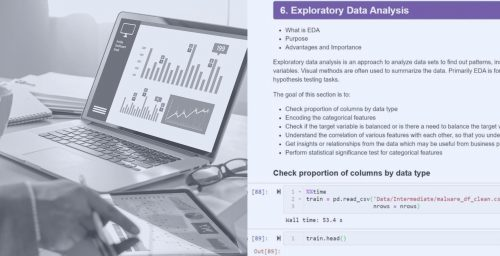Measures of Central Tendency

Measures of Dispersion

Correlation and Correlation Test

Chi-Squared Test

ANOVA

Types of missing values

Identify missing values

Visualise missing values using 'missingo'

Dropping rows or columns with missing values (how and when)

Replace with 0, mean, median, mode, most frequent, string

Causes of missing values and when simple methods fail?

Predict the missing value

How to Validate the Accuracy of Your Missing Value Treatment Approach

How to spot Outliers- Box plot interpretation - IQR

How to spot Outliers-Z score

Multivariate outliers- Multivariate

Multivariate outliers- Series

Local Outlier factor

Isolation forest

Feature Scaling

Feature Encoding

Standardization vs Normalization

Plotting Correlation: Relationship between Two Numerical Variables- Scatterplot and Lines of Best Fit

Plotting Correlation: Relationship between two numerical variables- Correlation matrix with Heatmap

Histogram and Density plot

Bar Plot, Stacked

Box Plot and Violin Plot

Multiple Box Plots

Bubble Plots

Pair Plots

Trellis Chart or Facets

Mosaic Plots

Line Plots

Line Plots with Multiple Lines

Line Plots with Secondary Axis

Autocorrelation Plot

Time Series Seasonal Plots- Multi-Line chart

Time Series Seasonal Plots- The monthly and yearly box plot

Waterfall Chart (How to Create and Where is it Used?)

3D Plots

Problem Statement

Data Overview

Univariate Analysis

Bivariate Analysis - Statistical Significance Tests

Problem Statement

Data Overview

Univariate Analysis

Bivariate Analysis - Statistical Significance Tests

Summary

Assignment/ Challenges

Certificate of completion

• Regression and Regularization
ML with Python   Launching Oct21

5h 34m 23s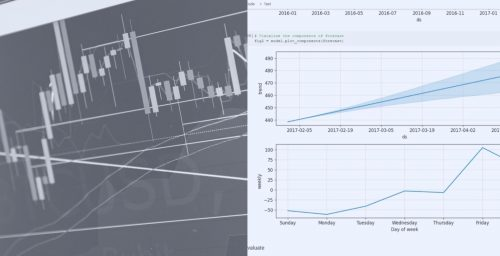Introduction to Linear Regression

Industrial Applications of Regression

Simple Linear Regression - Ordinary Least Square (OLS)

Multiple Linear Regression - Intuition

Cost Function

Model Diagnostics - R square and Adj R square

Model Diagnostics - p-value

Model Diagnostics - VIF

Model Diagnostics - AIC/BIC

Assumptions of Linear Regression

Mean Absolute Error

Mean Absolute Percentage Error

Mean Squared Error

Room Mean Squared Error

Problem Statement

Data Overview

EDA

Data Preprocessing

Various Approaches to implementing Linear Regression

Forward Stepwise Regression

Backward Stepwise Regression

Stepwise Regression

Sklearn Implementation

Model Evaluation

Check Assumptions of Linear regression

Intuition and Motivation

Polynomial Regression Implementation

How to Choose the Degree of the Polynomial

Underfitting and Overfitting

Cross-Validation

L1 Regularization Intuition

L2 Regularization Intuition

Elastic net Regularization Intuition

L1 Regularization Implementation

L2 Regularization Implementation

Elastic-net Regularization Implementation

Summary

Assignment/ Challenges

Certificate of completion

• Getting Started with Classification Algorithms
ML with Python   Launching Oct21

3h 10m 31s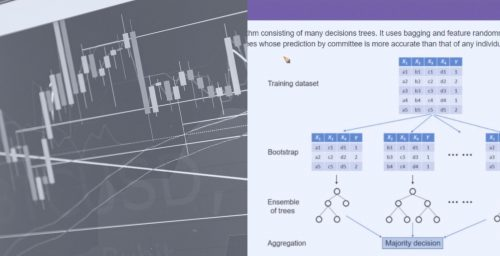Introduction to Logistic Regression

Industrial Applications of Logistic Regression/Classification

Solve Multiclass Classification using Logistic Regression

Graphical and Geometric Representation

The Logistic Function

Why use Logistic Regression over Linear Regression

Linear Regression to Logistic Regression

Maths behind Logistic Regression

Accuracy

Confusion Matrix - TP, TN, FP, FN

Classification Report - Precision, Recall, F1-score

ROC Curve

AUC

KS Statistic and Gain curve

Concordant and Discordant Pair

Problem Statement

Data Overview

EDA

Data Preprocessing

Forward Stepwise Regression

Model Implementation

Model Evaluation

L1 Regularization Implementation

L2 Regularization Implementation

Elastic-net Regularization Implementation

Summary

Assignment/ Challenges

Certificate of completion

• Handling Imbalanced Data for Classification
ML with Python   Launching Nov21

2h 25m 41s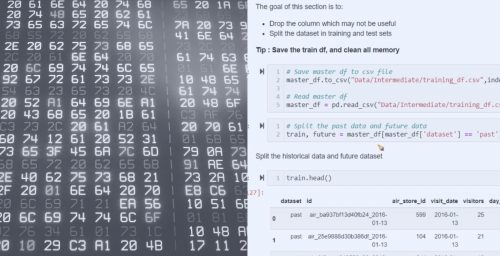What is imbalanced data

Why is an imbalanced data problem for classification

How to fix the problem of class imbalance

Upsampling minority class using resample

Undersampling majority class using resample

Random undersampling using imbalanced-learn

Random oversampling using imbalanced-learn

Oversampling using SMOTE

Oversampling followed by under-sampling

Using inbuilt class_wight parameter of algorithms

One-Class SVM

Mahalanobis Distance

LightGBM with the focal loss

Summary

Assignment/ Challenges

Certificate of completion

• Supervised Learning
ML with Python   Launching Nov21

5h 49m 23s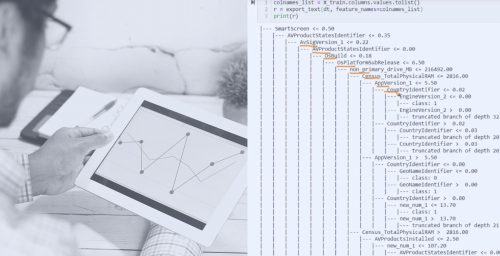Introduction to KNN algorithm

Intuition behind KNN

Maths behind KNN

How to choose the optimal value for k

Problem Statement

Data Overview and Preprocessing

Model Building

Model Processing

Model Refinement Using Different Values for k

Hyperparameter tuning to find the best value of k

Introduction to Naive Bayes algorithm

The Intuition behind Naive Bayes

Various Types of Naive Bayes

Problem Statement

Data Overview and Preprocessing

Model Building - Gaussian Naive Bayes

Model Building - Bernoulli Naive Bayes

Model Evaluation

Introduction to SVM Algorithm

The Intuition Behind SVM Classification

Maths Behind SVM

Various Kernels of SVM

Kernel Tricks

Problem Statement

Data Overview and Preprocessing

Model Building

Model Evaluation

Model Refinement Using Different Kernels

Model Improvement Using Hyperparameter Tuning

Problem Statement

Data Overview and Preprocessing

Model Building

Model Evaluation

Model Refinement using Different Kernels

Introduction to Decision Tree Algorithm

The Intuition Behind Decision Tree Classification

The Intuition Behind Decision Tree Regression

The Measure of Impurity Classification

The Measure of Impurity Regression

How to Visualize and Interpret Decision Tree

Problem Statement

Data Overview and Preprocessing

Model Building

Model Evaluation

Model Refinement using Different Kernels

Summary

Assignment/ Challenges

Certificate of completion

• Ensemble Learning
ML with Python   Launching Dec21

5h 39m 07s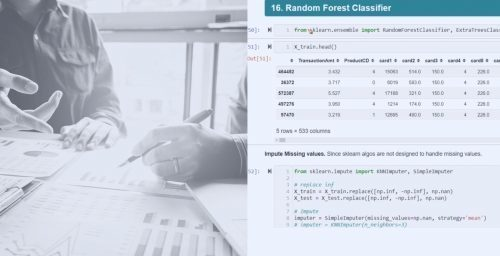What is Ensemble Learning

Bagging vs Boosting

Evolution of Boosting Algorithms

Introduction to Random Forest

The Intuition Behind Random Forest

Maths Behind Random Forest

Bootstrap Aggregation

Various parameters of random forest

Problem Statement

Data Overview and Preprocessing

Model Building

Model Refinement using various parameters

Hyperparameter tuning

Problem Statement

Data Overview and Preprocessing

Model Building

Model Evaluation

Model Refinement using various parameters

Hyperparameter tuning

Introduction to CatBoost

The intuition behind CatBoost

Maths behind CatBoost

Various parameters of CatBoost

Problem Statement

Data Overview and Preprocessing

Model Building

Model Evaluation

Model Refinement using various parameters

Hyperparameter tuning

The Intuition Behind XGBoost

Problem Statement

Data Overview and Preprocessing

Model Building

Model Evaluation

Model Refinement using various parameters

Hyperparameter tuning

Introduction to LightGBM

The intuition behind LightGBM

Maths behind LightGBM

Various parameters of LightGBM

Problem Statement

Data Overview and Preprocessing

Model Building

Model Evaluation

Model Refinement using various parameters

Hyperparameter tuning

Final Words (2:45)

Assignment/ Challenges

Certificate of completion

• Feature Engineering
ML with Python   Launching Dec21

5h 02m 31s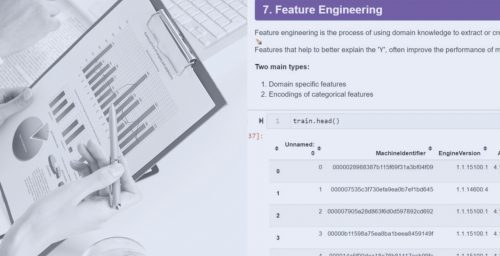Overview of Numerical data

Feature Standardization

Feature Normalization

Feature Binning

Feature Transformation

Feature Interactions

Ranks

Splines

Overview of Categorical data

Feature Encoding

Dummy Coding

Contrast Coding

Bin Counting

Feature Hashing

Feature Interactions

Overview of Text data

Count Vectors

Tf-idf Vectors

Work Tokenization

Bag of Words

Stemming

Lemmatization

POS Tagging

Reduce Dimensions using Feature Hashing

Overview of Time Series data

Date related feature engineering

Time-related feature engineering

Lag features

Sliding window features

Rolling window features

Expanding window features

Feature Interactions

Summary

Assignment/ Challenges

Certificate of completion

• Feature Selection
ML with Python   Launching Jan22

3h 13m 34s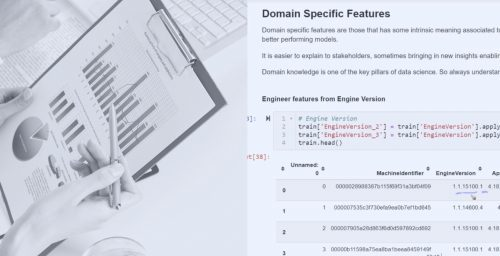What is Feature Selection

Why Feature Selection

Types of Feature Selection Algorithm

Pearson Correlation Intuitions

Pearson Correlation Implementation

LDA Intuitions

LDA Implementation

ANOVA Intuitions

ANOVA Implementation

Chi-square Intuitions

Chi-square Implementation

Forward Selection Intuitions

Forward Selection Implementation

Backward Selection Intuitions

Backward Selection Implementation

Stepwise Selection Intuitions

Stepwise Selection Implementation

Recursive Features Selection Intuitions

Recursive Feature Selection Implementation

Lasso Regression Intuitions

Lasso Regression Implementation

Random Forest Intuitions

Randorm Forest Implementation

Summary

Assignment/ Challenges

Certificate of completion

• Dimensionality Reduction
ML with Python   Launching Jan22

4h 55m 23s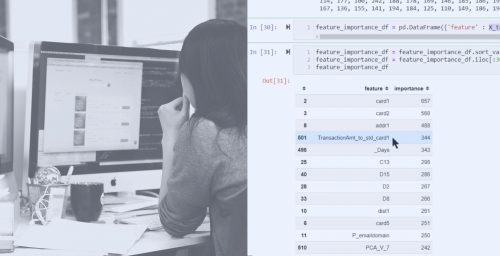Curse of Dimensionality

How Dimensionality Reduction Solves the Problem

Intuitions Behind PCA

Maths Behind PCA

PCA Implementation

Intuitions Behind t-SNE

Maths Behind t-SNE

T-SNE Implementation

Intuitions Behind Sammon mapping

Maths behind Sammon mapping

Sammon mapping implementation

Intuitions behind Self Organizing Maps

Maths behind Self Organizing Maps

Maths behind Self Organizing Maps

Intuitions behind LDA

Maths behind LDA

LDA Implementation

Intuitions Behind MDS

Maths Behind MDS

MDS Implementation

Intuitions Behind ICA

Maths Behind ICA

ICA Implementation

Intuitions Behind Non-Negative Matrix Factorization

Maths Behind Non-Negative Matrix Factorization

Non-Negative Matrix Factorization implementation

Summary

Assignment/ Challenges

Certificate of completion

• Deployment of ML Models
ML with Python   Launching Jan22

9h 48m 23s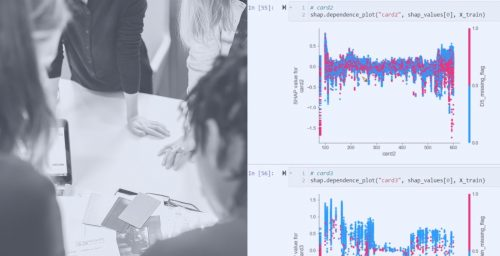Deployments of Machine Learning Model

Deployment of Machine Learning Pipelines

Research and Production Environment

Building Reproducible Machine Learning Pipelines

Challenges to Reproducibility

Streamlining Model Deployment with Open-Source

Machine Learning System Architecture and Why it Matters

Specific Challenges of Machine Learning Systems

Principles for Machine Learning Systems

Machine Learning System Architecture Approaches

Machine Learning System Component Breakdown

Research Environment - Process Overview

Machine Learning Pipeline Overview

Feature Engineering - Variable Characteristics

Feature Engineering Techniques

Feature Selection

Training a Machine Learning Model

Data Analysis Demo - Missing Data

Data Analysis Demo - Temporal Variables

Data Analysis Demo - Numerical Variables

Data Analysis Demo - Categorical Variables

Feature Engineering Demo 1

Feature Engineering Demo 2

Feature Selection Demo

Model Training Demo

Scoring New Data With Our Model

Python Open Source for Machine Learning

Open Source Libraries for Feature Engineering

Feature Engineering with Open Source Demo

Intro to Object Oriented Programing

Inheritance and the Scikit-learn API

Create Scikit-Learn Compatible Transformers

Create Transformers that Learn Parameters

Feature Engineering Pipeline Demo

Should Feature Selection be Part of the Pipeline?

Getting Ready for Deployment - Final Pipeline

Introduction to Production Code

Code Overview

Package Requirements Files

Working with tox

Package Config

The Model Training Script & Pipeline

Introduction to Pytest [Optional]

Feature Engineering Code in the Package

Making Predictions with the Package

Building the Package

Tooling

Running the API Locally

Understanding the Architecture of the API

Introduction to FastAPI

The API Endpoints

Using Schemas in our API

Logging in our Application

The Uvicorn Web Server

Introducing Heroku and Platform as a Service (PaaS)

Deploying our Application to Heroku

Understanding the Heroku-Specific Project Files

Introduction to CI/CD

Setting up CircleCI

CI/CD Automation Overview Part 1

CI/CD Config

CI/CD Automation Overview Part 2

Using a Private Index Server (Gemfury)

Hands on: Run the CI Tests in your own Github Fork

Hands on: Run the CI Deploy on Your Own Github Fork

Hands on: Run the CI Publish on Your Own Github Fork

Docker Refresher [Optional - For those unfamiliar/rusty with Docker]

The Value of Docker and Containers

Understanding The Container Deployment Process

Hands On: Containerising the App Locally

Updating the CI Pipeline for a Container Deployment

How to Use the Course Resources

Introduction

Setting up Differential Tests

Differential Tests in CI (Part 1 of 2)

Differential Tests in CI (Part 2 of 2)

Wrap up

Introduction to AWS

AWS Costs and Caution

Ntro to AWS ECS

Container Orchestration Options: Kubernetes, ECS, Docker Swarm

Create an AWS Account

Setting Permissions with IAM

Installing the AWS CLI

Configuring the AWS CLI

Intro the Elastic Container Registry (ECR)

Creating the ECS Cluster with Fargate Launch Method

Creating the ECS Cluster with the EC2 Launch Method

Updating the Cluster Containers

Tearing down the ECS Cluster

Deploying to ECS via the CI pipeline

Wrap up

Challenges of using Big Data in Machine Learning

Introduction to a Large Dataset - Plant Seedlings Images

Building a CNN in the Research Environment

Production Code for a CNN Learning Pipeline

Reproducibility in Neural Networks

Packaging the CNN

Adding the CNN to the API

Introduction

Primer on Monorepos

Creating the API Skeleton

API Schema Validation

Wrap Up

Summary

Assignment/ Challenges

Certificate of completion

• Unsupervised Learning
ML with Python   Launching Feb22

6h 09m 23s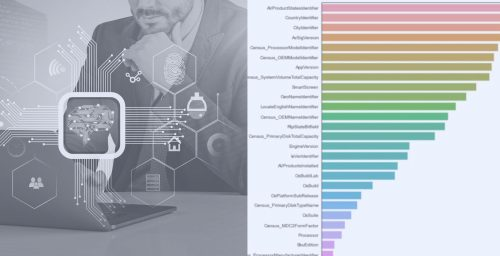What is unsupervised learning

Industrial Applications of unsupervised learning

Intuitions behind k-means

Maths behind k-means

How to select the optimal number of clusters

K-means ++

Problem Statement

EDA and data preprocessing

Model Building

Find the optimal number of clusters

Visualize the clusters

Intuitions behind k-medoids

Maths behind k-medoids

Problem Statement

EDA and data preprocessing

Model Building

Visualize the clusters

Intuitions behind hierarchical clustering

Maths behind hierarchical clustering

Visualize the hierarchical clustering model

Problem Statement

EDA and data preprocessing

Model Building

Visualize the clusters

Intuitions behind DBSCAN clustering

Maths behind DBSCAN clustering

Problem Statement

EDA and data preprocessing

Model Building

Visualize the clusters

Intuitions behind fuzzy-c clustering

Maths behind fuzzy-c clustering

Problem Statement

EDA and data preprocessing

Model Building

Visualize the clusters

Intuitions behind GMM

Maths behind GMM

Problem Statement

EDA and data preprocessing

Model Building

Visualize the clusters

Summary

Assignment/ Challenges

Certificate of completion

• Recommendation System
ML with Python   Launching Feb22

5h 18m 29s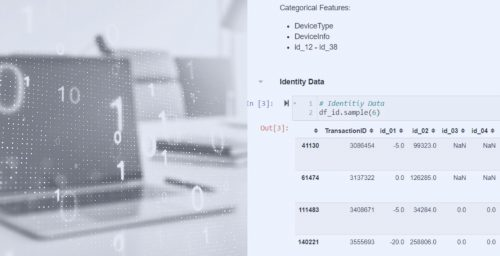Getting the most from this course

What Is a Recommender System?

Types of recommenders

Understanding You through Implicit and Explicit Ratings

Top-N Recommender Architecture

Ranks

[Activity] The Basics of Python

Data Structures in Python

Functions in Python

[Exercise] Booleans, loops, and a hands-on challenge

Train/test and cross validation

Accuracy Metrics (RMSE, MAE)

Top-N Hit Rate - Many Ways

Coverage, Diversity, and Novelty

Churn, Responsiveness, and A/B Tests

[Quiz] Review ways to measure your recommender.

[Activity] Walkthrough of RecommenderMetrics.py

Walkthrough of test metrices

Measure the Performance of SVD Recommendations

Our Recommender Engine Architecture

[Activity] Recommender Engine Walkthrough, Part 1

[Activity] Recommender Engine Walkthrough, Part 2

[Activity] Review the Results of our Algorithm Evaluation.

Content based recommendations and the cosin similarity metrics

K-Nearest-Neighbors and Content Recs

[Activity] Producing and Evaluating Content-Based Movie Recommendations

A Note on Using Implicit Ratings.

[Activity] Bleeding Edge Alert! Mise en Scene Recommendations

[Exercise] Dive Deeper into Content-Based Recommendations

Measuring Similarity and Sparsity

Similarity Metrics

User based collaborative Filtering

Preview

[Activity] User-based Collaborative Filtering, Hands-On

Item-based Collaborative Filtering

[Activity] Item-based Collaborative Filtering, Hands-On

[Exercise] Tuning Collaborative Filtering Algorithms

[Activity] Evaluating Collaborative Filtering Systems Offline

[Exercise] Measure the Hit Rate of Item-Based Collaborative Filtering

KNN Recommenders

[Activity] Running User and Item-Based KNN on MovieLens

[Exercise] Experiment with different KNN parameters.

Principal component analysis

Singular Value Decomposition

Preview

Improving on SVD

[Exercise] Tune the Hyperparameters on SVD

Bleeding Edge Alert! Sparse Linear Methods (SLIM)

Deep Learning Introduction

Deep Learning Pre-Requisites

History of Artificial Neural Networks

[Activity] Playing with Tensorflow

Training Neural Networks

Tuning Neural Networks

Activation Functions: More Depth

Introduction to Tensorflow

[Activity] Handwriting Recognition with Tensorflow, part 1

[Activity] Handwriting Recognition with Tensorflow, part 2

Introduction to Keras

[Activity] Handwriting Recognition with Keras

Classifier Patterns with Keras

[Exercise] Predict Political Parties of Politicians with Keras

Intro to Convolutional Neural Networks (CNN's)

CNN Architectures

[Activity] Handwriting Recognition with Convolutional Neural Networks (CNNs)

Intro to Recurrent Neural Networks (RNN's)

Training Recurrent Neural Networks

[Activity] Sentiment Analysis of Movie Reviews using RNN's and Keras

Tuning Neural Networks

GAN's in Action

Generating Images of Clothing with Generative Adversarial Networks

Intro to Deep Learning for Recommenders

[Activity] Recommendations with RBM's, part 1

[Activity] Recommendations with RBM's, part 2

[Activity] Evaluating the RBM Recommender

[Exercise] Tuning Restricted Boltzmann Machines

Exercise Results: Tuning a RBM Recommender

Auto-Encoders for Recommendations: Deep Learning for Recs

[Activity] Recommendations with Deep Neural Networks

Clickstream Recommendations with RNN's

[Exercise] Get GRU4Rec Working on your Desktop

Exercise Results: GRU4Rec in Action

Tensorflow Recommenders (TFRS): Building a Ranking Stage

TFRS: Incorporating Side Features and Deep Retrieval

TFRS: Multi-Task Recommenders, Deep & Cross Networks, ScaNN, and Serving

Bleeding Edge Alert! Deep Factorization Machines

More Emerging Tech to Watch

Introduction and Installation of Apache Spark

Apache Spark Architecture

[Activity] Movie Recommendations with Spark, Matrix Factorization, and ALS

[Activity] Recommendations from 20 million ratings with Spark

DSSTNE in Action

Scaling Up DSSTNE

AWS SageMaker and Factorization Machines

SageMaker in Action: Factorization Machines on one million ratings, in the cloud

Other Systems of Note (Amazon Personalize, RichRelevance, Recombee, and more)

Recommender System Architecture The Cold Start Problem (and solutions)

[Exercise] Implement Random Exploration

Exercise Solution: Random Exploration

[Exercise] Implement a Stoplist

Exercise Solution: Implement a Stoplist

[Exercise] Identify and Eliminate Outlier Users

Exercise Solution: Outlier Removal

Fraud, The Perils of Clickstream, and International Concerns

Temporal Effects, and Value-Aware Recommendations

Case Study: Netflix, Part 1

Case Study: Netflix, Part 2

Hybrid Recommenders and Exercise

Exercise Solution: Hybrid Recommenders

More to Explore

Summary

Assignment/ Challenges

Certificate of completion

##### Course reviews

My students have published novel research papers, changed their careers from developers to computer vision/deep learning practitioners, successfully applied CV/DL to their work projects, landed positions at R&D companies, and won grant/award funding for research. Take a look and see for yourself how PyImageSearch University can help you in your journey.

4.84 Based on 128 Reviews
• 5 stars

%

• 4 stars

%

• 3 stars

%

• 2 stars

%

• 1 star

%

PyImageSearch University is really the best Computer Visions “Masters” Degree that I wish I had when starting out. Being able to access all of Adrian’s tutorials in a single indexed page and being able to start playing around with the code without going through the nightmare of setting up everything is just amazing. 10/10 would recommend.##### Sanyam Bhutani
Machine Learning Engineer and 2x Kaggle Master

Not going to kid you: PyImageSearch University is worth every cent. I get asked ALL the time at my talks how I got started. PyImageSearch was the foundation.##### Paul Zikopoulos
IBM VP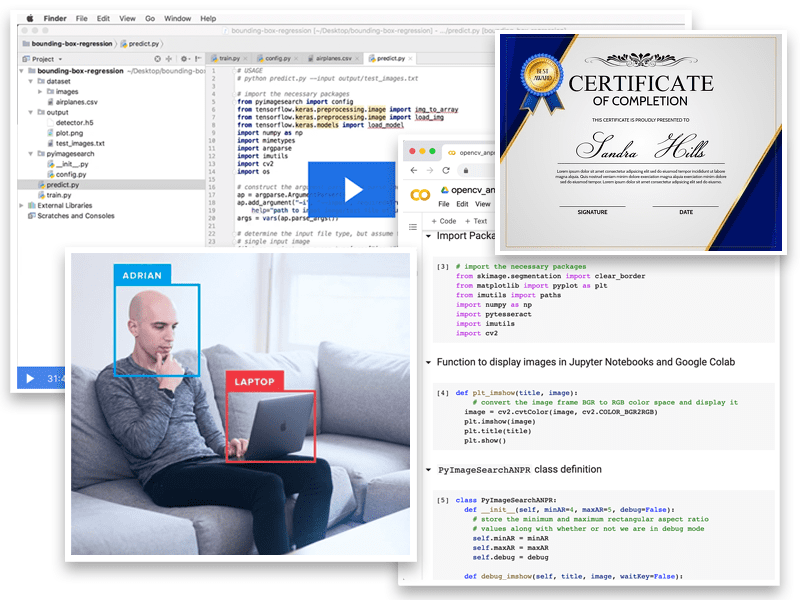No commitments, cancel anytime.

This course includes:

Brand new courses released every month, ensuring you can keep up with state-of-the-art techniques

37h 19m on-demand video

25 courses on essential computer vision, deep learning, and OpenCV topics

25 Certificates of Completion

Pre-configured Jupyter Notebooks in Google Colab for 200+ PyImageSearch tutorials

Access on mobile, laptop, desktop, etc.

##### I already have a PyImageSearch University account. How do I login?

Thank you for being a member of PyImageSearch University! You can login here.

##### Do I need any programming experience before joining PyImageSearch University?

We assume you have some prior programming experience (e.g. you know what a variable, function, loop, etc. are). You should have more skills than a novice, but certainly not an intermediate or advanced developer. As long as you understand basic programming logic flow you’ll be successful inside PyImageSearch University.

##### Do I need to know anything about computer vision, deep learning, or OpenCV to get started in PyImageSearch University?

No. The courses inside PyImageSearch University will teach you computer vision, deep learning, and OpenCV. As long as you have basic programming experience you will be successful inside PyImageSearch University.

##### Do I need any special software or hardware?

No. All of our courses, coding exercises, etc. can be completed inside your browser using our pre-configured Jupyter Notebooks running in Google Colab. If you prefer to instead configure your local development environment, we provide install instructions as well.

##### How will I be charged?

For monthly and yearly memberships, you will be charged on a recurring monthly or yearly basis, depending on your subscription type, starting from the sign-up date. You can cancel at any time.

#### Hi there — I’m Adrian Rosebrock.

Hey, I’m Adrian Rosebrock, a Ph.D and entrepreneur who has spent his entire adult life studying computer vision and machine learning. Over the past 6 years alone I have:

• Started the PyImageSearch.com blog and published over 400+ tutorials and articles aimed at teaching computer vision, deep learning, and OpenCV.
• Authored Deep Learning for Computer Vision with Python, the most in-depth computer vision and deep learning book available today, including super practical walkthroughs, hands-on tutorials (with lots of code), and a no-nonsense teaching style that will help you master computer vision and deep learning.
• Answered over 50,000+ emails and helped 10,000s of developers, researchers, and students just like yourself learn the ropes of computer vision and deep learning.

If having an actual PhD in the field teach you computer vision, deep learning, and OpenCV sounds interesting to you, join me inside PyImageSearch University. You’ll learn a ton about computer vision and deep learning in a practical, hands-on way. And you’ll have fun doing it. See you on the other side!Course Preview

## Machine Learning A-Z™: Hands-On Python & R In Data Science

### Free Sample Videos:#### Machine Learning A-Z™: Hands-On Python & R In Data Science#### Machine Learning A-Z™: Hands-On Python & R In Data Science#### Machine Learning A-Z™: Hands-On Python & R In Data Science#### Machine Learning A-Z™: Hands-On Python & R In Data Science